Synergetics. Etudes 70.

Is Dedicated to Centennial of Burthday of Outstanding Scientist, Professor

Basin Abram M.

Basina G. I., Basin M. A.

SIC ”Synergetics” of Saint-Petersburg Association of Scientist and Scholars.

Etude 4.

Many-dimensional Power Complex Systems of Iterative Relations. Exponent of Circumference as One of Forms of Fundamental Decision of the System.

December 18, 2009.

At the decision of nonlinear many-dimensional problems of dynamics of complex systems essentially role play complex operators, which may be called power operators. We consider many-dimensional iterative symbolic relation: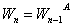.                                                               (1)

Here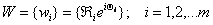- aggregate of spiral complex coordinates;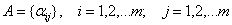(2)

is the linear complex operator, which elements are algebraic complex numbers (See Etude5).

Relation (1) is symbolic representation of formula: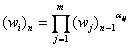.                                                         (3)

We take logarithm from left and right parts of equality (3)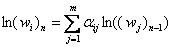,                                                     (4)

or in operator form –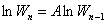.                                                         (5)

We introduce the next designation: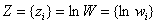.                                                   (6)

Then equation (5) will be written in the form: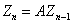,-                                                             (7)

and the equality(4) – in the form: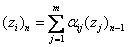.                                                        (8)

We introduce complex finite-dimensionally affine complex vector space. As the vector in our complex space we shall consider the aggregate of complex numbers; transforming for the relation (8)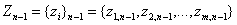.                                           (9)

We consider, that the space is related to determined orts: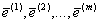.                                                  (10)

So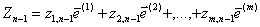.                                    (11)

We call equalities (7), (8) as linear transformation of vector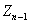to the vector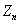.

If the determinant of matrix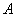is not equal to zero, then solving equations (7), (8) relativelywe receive: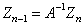,                                                 (12)

where matrix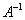has elements: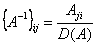.                                                             (13)

Here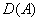is determinant of matrix, and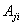are its algebraic addendums in relation to elements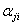.We introduce new orts: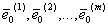.                                                          (14)

Connection between the new and old orts expresses with relations: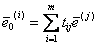.                                                    (15)

Determinant of matrix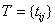(16)

is not equal to zero. If any vectorin system of coordinates with orts (10) had the components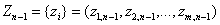, then in new coordinates system it will have another components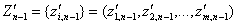,                                  (17)

which express through the first with help of relations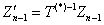.                                             (18)

Here the matrix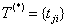is transposed relative. If we have any iterative process, which in primary coordinate system was expressed with formula (7), then in the new coordinate system the same process will be expressed with the formula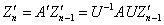,                                                    (18)

where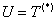.                                                  (19)

Matrix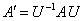(20)

is called similar to the matrix. Similar matrixes are equivalent in that relation, that they determine the same transformation, but represented in different systems of coordinates. If the determinant of matrixis not equal to zero, then through the matrixes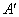are diagonal matrixes , for which are not equal to zero only diagonal members. In the case of choice of transformation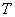with such a matter, that matrixwill be diagonal matrix, then relation (18) will transform in complex one-dimensional iteration relations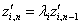,                                                       (21)

where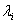-are diagonal members of matrix..                                                        (22)

For the finding of corresponding matrixand diagonal members of matrixwe write the next equality: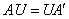,-                                                           (23)

or in the coordinate form: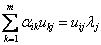.                                                    (24)

If we take the elements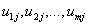as components of any vector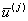, then we can write the equality (24)in the form of vector equality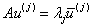.                                              (25)

«So we see, that finding of matrix, which reduces the matrixto the diagonal form consists in finding such vectors, which reproduce with the accuracy of complex number factor as a result of linear transformation, determining by matrix. This fact is algebraic analog of that fact of contemporary quantum mechanics, according with which the matrix mechanics of Heisenberg is equivalent to wave mechanics of Schrödinger. According to the first point of view essential problem is the transformation of any matrix (infinite) to the diagonal form. As concerns the wave  mechanics, here essential question is the problem of finding such vectors (in the space with infinite number of dimensions), which reproduce with the accuracy of number factors the result of any linear transformation. Former considerations we called algebraic analog that’s why, when we bound with finite number of dimensions, we lead our problems to pure algebraic problems. In more complex cases with infinite variety of dimensions we go out from the frames of usual algebra and we need to use the apparatus of analysis .

System (24)or (25) may be written in the form: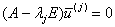.                                                   (26)

Here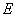is single matrix. For becoming of the decision, which is not equal to zero the determinant of matrix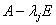must be equal to zero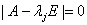.                                            (27)

We received characteristic complex algebraic equation of the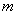- order, which has equalcomplex decisions. Suppose that these decisions are different. So we find all meanings of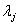and may to build the matrix,                                            (28)

and instead of iteration system (12) to decide the one-dimensionally iterative relations (21), which we  have considered in Etude №3. We must now to determine with the accuracy of any complex multiplier not equal to zero the elements of matrix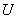. In the system (26) we take any meaning of one of coordinates of vector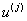and transfer any members from left part in the right part of the system (26), excluding one of equations. If receiving in this case multivendor system of equations has determinant not equal to zero, than its decision determines with formula of Kramer . This procedure repeats for all meanings of. We return to the system of iterative relations (21). Substituting consecutive in the right part the meanings of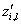as far as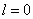, we receive: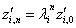.                                                   (29)

Equality(29)determines fundamental decision of system (21).We introduce new complex varieties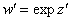.                                                       (30)

Then the system (29) will be written in the form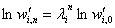.                                             (31)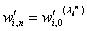.                                                (32)

Ifhas the modulus, equal to 1, than the meanings of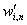for all whole meanings of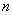lay on the exponent of circumference (see Etudes 1-3). We may introduce the exponential vector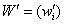.                                                   (33)

Then the relation (32) may be written in symbolic form: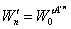.                                                    (34)

Relation (31) may be written in the form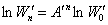(35)

Last relation is written in the term of affine vectors and classical matrixes. Shall we return in relation (35) to primary coordinate system, using the relations (18)-(20)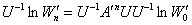.                                         (36)

Or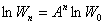.                                                            (37)

Exponentiating  (37), we receive symbolic decision of system (1)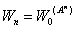.                                                            (38)

Between coordinates of exponential vector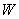realizes the connection according the relation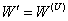,                                                                (39)

or in coordinate form –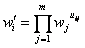(40)

Literature.

1. Smirnov V. I. Curse of High Mathematics for Techniques and Physicists. B. 3 “GITTI”M.-_L.. 1933. 736 p.